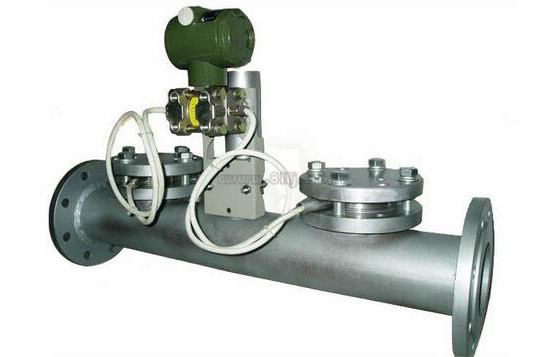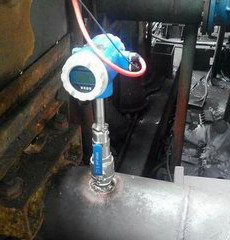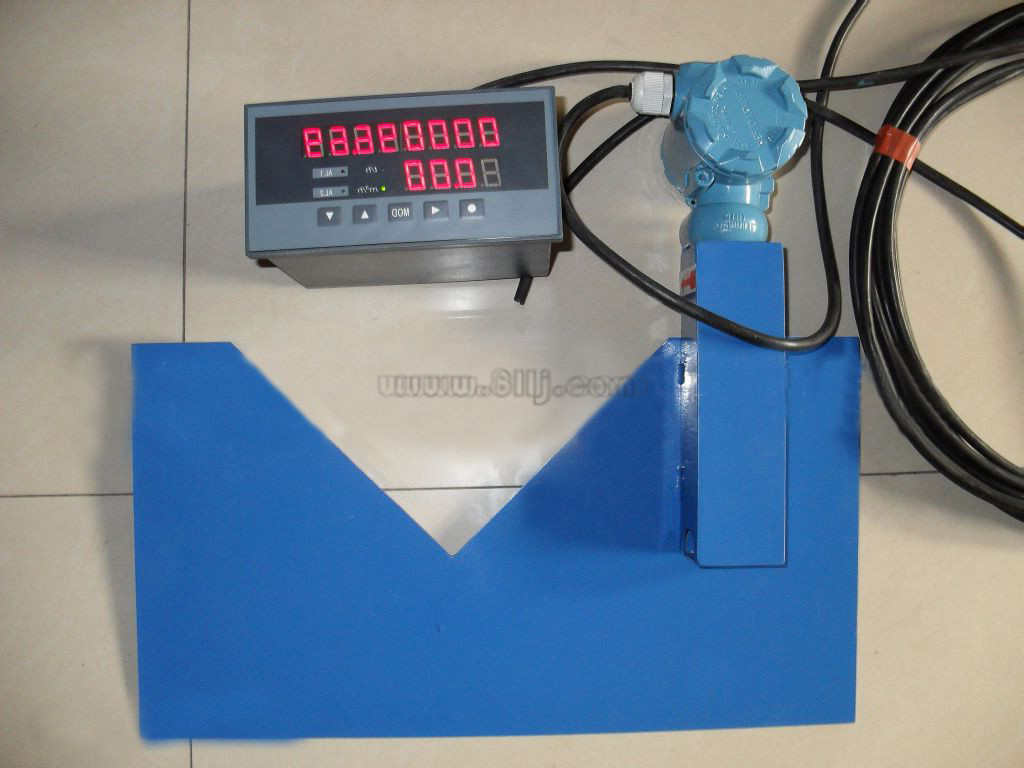15222906608 微信同号# 核电站一回路稳压器水位测量与计算

△P0=P+-P_=[ρ3g（L3+L4）+ρ5gL5]-（ρ1gL1+ρ2gL2）

△P0=0.10l9×lO3×9.8×（O.013+9.765）十1.0O2×1O3×9.8×1.938-1.0O2×lO3×9.8×0.341

-1.002×1O3×9.8×11.375=-86251.54

△P100%=P+-P_=（ρ3gL3十ρ4gL4十ρ5gL5）-（ρ1gL1十ρ2gL2）=0.10l9×lO3×9.8×0.013十0.59395×103×9.8×9.765+1.002×103×9.8×1.938一1.002×1O3×9.8×0，341-1.002×1O3×9.8×11.375=-39163.83

P_=ρ×g×（L1十L2）而ρ=l000.54-0.0252×t-O.0045×t2

p=1000.54-0.0252×25-0.0045×252=997.0975kg/m3

p_=997.0975×9.8×11.716=114483.554

△Po=P+o-P-

P+o=28232

△Pl00%=P+l00%—P—

P+l00%=75315.9

1O3kg/m3，而对在其它温度时水的密度，可以查表或根据经验公式p=l000.54-0.0252×t-O.0045×t2计算。《核电站一回路稳压器水位测量与计算》相关新闻皖公网安备 34118102000583号